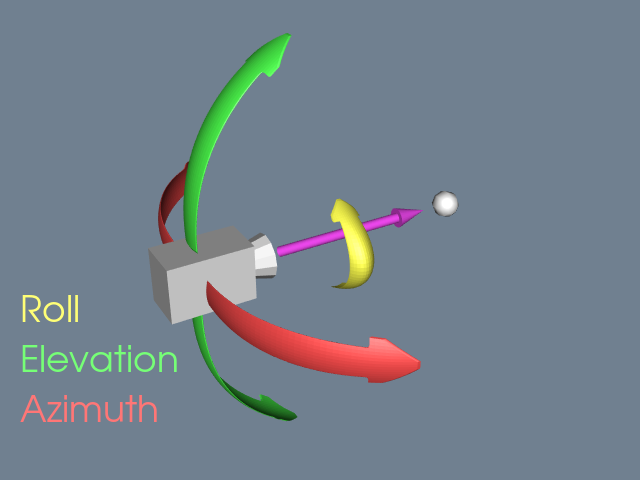# Cameras¶

The `pyvista.Camera` class adds additional functionality and a pythonic API to the `vtk.vtkCamera` class. `pyvista.Camera` objects come with a default set of cameras that work well in most cases, but in many situations a more hands-on approach to using the camera is necessary.

## Brief Example¶

Create a frustum of camera, then create a scene of inside frustum.

```import pyvista as pv
import numpy as np
import vtk
from pyvista import examples

pv.set_plot_theme("document")

camera = pv.Camera()
near_range = 0.3
far_range = 0.8
camera.clipping_range = (near_range, far_range)
unit_vector = np.array(camera.direction) / np.linalg.norm(
np.array([camera.focal_point]) - np.array([camera.position])
)

frustum = camera.view_frustum(1.0)

position = camera.position
focal_point = camera.focal_point
line = pv.Line(position, focal_point)

xyz = camera.position + unit_vector * 0.6 - np.mean(bunny.points, axis=0)
bunny.translate(xyz)

pl = pv.Plotter(shape=(2, 1))
pl.subplot(0, 0)
[
position,
camera.position + unit_vector * near_range,
camera.position + unit_vector * far_range,
focal_point,
],
["Camera Position", "Near Clipping Plane", "Far Clipping Plane", "Focal Point"],
margin=0,
fill_shape=False,
font_size=14,
shape_color="white",
point_color="red",
text_color="black",
)
pl.camera.position = (1.1, 1.5, 0.0)
pl.camera.focal_point = (0.2, 0.3, 0.3)
pl.camera.up = (0.0, 1.0, 0.0)
pl.camera.zoom(1.4)

pl.subplot(1, 0)
pl.camera = camera
pl.show()
```

## Controlling Camera Rotation¶

In addition to directly controlling the camera position by setting it via the `pyvista.Camera.position` property, you can also directly control the `pyvista.Camera.roll`, `pyvista.Camera.elevation`, and `pyvista.Camera.azimuth` of the camera.For example, you can modify the roll. First, generate a plot of an orientation cube while initially setting the camera position to look at the `'yz'`.

```import pyvista
from pyvista import demos
pl = demos.orientation_plotter()
pl.camera_position = 'yz'
pl.show()
```

Here we modify the roll in-place.

```import pyvista
from pyvista import demos
pl = demos.orientation_plotter()
pl.camera_position = 'yz'
pl.camera.roll += 10
pl.show()
```

And here we offset the azimuth of the camera by 45 degrees to look at the `X+` and `Y+` faces.

```import pyvista
from pyvista import demos
pl = demos.orientation_plotter()
pl.camera_position = 'yz'
pl.camera.azimuth = 45
pl.show()
```

Here, we move upward by setting the elevation of the camera to 45 degrees to see the `X+` and `Z+` faces.

```import pyvista
from pyvista import demos
pl = demos.orientation_plotter()
pl.camera_position = 'yz'
pl.camera.elevation = 45
pl.show()
```

### API reference¶

class `pyvista.``Camera`

PyVista wrapper for the VTK Camera class.

Examples

Create a camera at the pyvista module level

```>>> import pyvista
>>> camera = pyvista.Camera()
```

Access the active camera of a plotter and get the position of the camera.

```>>> pl = pyvista.Plotter()
>>> pl.camera.position
(1.0, 1.0, 1.0)
```
property `azimuth`

Azimuth of the camera.

Rotate the camera about the view up vector centered at the focal point. Note that the view up vector is whatever was set via SetViewUp, and is not necessarily perpendicular to the direction of projection.

Examples

```>>> import pyvista
>>> pl = pyvista.Plotter()
>>> pl.camera.azimuth
0.0
```
property `clipping_range`

Return the location of the near and far clipping planes along the direction of projection.

Examples

```>>> import pyvista
>>> pl = pyvista.Plotter()
>>> pl.camera.clipping_range
(0.01, 1000.01)
```
property `direction`

Vector from the camera position to the focal point.

Examples

```>>> import pyvista
>>> plotter = pyvista.Plotter()
>>> plotter.camera.direction
(0.0, 0.0, -1.0)
```
`disable_parallel_projection`()

Disable the use of perspective projection.

property `distance`

Distance from the camera position to the focal point.

Examples

```>>> import pyvista
>>> pl = pyvista.Plotter()
>>> pl.camera.distance
1.732050807568
```
property `elevation`

Vertical rotation of the scene.

Rotate the camera about the cross product of the negative of the direction of projection and the view up vector, using the focal point as the center of rotation.

Examples

```>>> import pyvista
>>> pl = pyvista.Plotter()
>>> pl.camera.elevation
0.0
```
`enable_parallel_projection`(flag=True)

Enable parallel projection.

The camera will have a parallel projection. Parallel projection is often useful when viewing images or 2D datasets.

property `focal_point`

Location of the camera’s focus in world coordinates.

Examples

```>>> import pyvista
>>> pl = pyvista.Plotter()
>>> pl.camera.focal_point
(0.0, 0.0, 0.0)
```
property `is_parallel_projection`

Return True if parallel projection is set.

property `model_transform_matrix`

Return the camera’s model transformation matrix.

Examples

```>>> import pyvista
>>> pl = pyvista.Plotter()
>>> pl.camera.model_transform_matrix
array([[1., 0., 0., 0.],
[0., 1., 0., 0.],
[0., 0., 1., 0.],
[0., 0., 0., 1.]])
```
property `parallel_scale`

Scaling used for a parallel projection.

Examples

```>>> import pyvista
>>> pl = pyvista.Plotter()
>>> pl.camera.parallel_scale
1.0
```
property `position`

Position of the camera in world coordinates.

Examples

```>>> import pyvista
>>> pl = pyvista.Plotter()
>>> pl.camera.position
(1.0, 1.0, 1.0)
```
property `roll`

Rotate the camera about the direction of projection.

This will spin the camera about its axis.

Examples

```>>> import pyvista
>>> pl = pyvista.Plotter()
>>> pl.camera.roll
-120.00000000000001
```
property `thickness`

Return the distance between clipping planes.

Examples

```>>> import pyvista
>>> pl = pyvista.Plotter()
>>> pl.camera.thickness
1000.0
```
property `up`

Return the “up” of the camera.

Examples

```>>> import pyvista
>>> pl = pyvista.Plotter()
>>> pl.camera.up
(0.0, 0.0, 1.0)
```
property `view_angle`

Return the camera view angle.

Examples

```>>> import pyvista
>>> plotter = pyvista.Plotter()
>>> plotter.camera.view_angle
30.0
```
`view_frustum`(aspect=1.0)

Get the view frustum.

Parameters

aspect (float, optional) – The aspect of the viewport to compute the planes. Defaults to 1.0.

Returns

frustum – View frustum.

Return type

pv.PolyData

Examples

```>>> import pyvista
>>> plotter = pyvista.Plotter()
>>> frustum = plotter.camera.view_frustum(1.0)
>>> frustum.n_points
8
>>> frustum.n_cells
6
```
`zoom`(value)

Set the zoom of the camera.

In perspective mode, decrease the view angle by the specified factor.

In parallel mode, decrease the parallel scale by the specified factor. A value greater than 1 is a zoom-in, a value less than 1 is a zoom-out.

Examples

```>>> import pyvista
>>> pl = pyvista.Plotter()
>>> pl.camera.zoom(2.0)
```